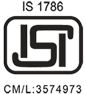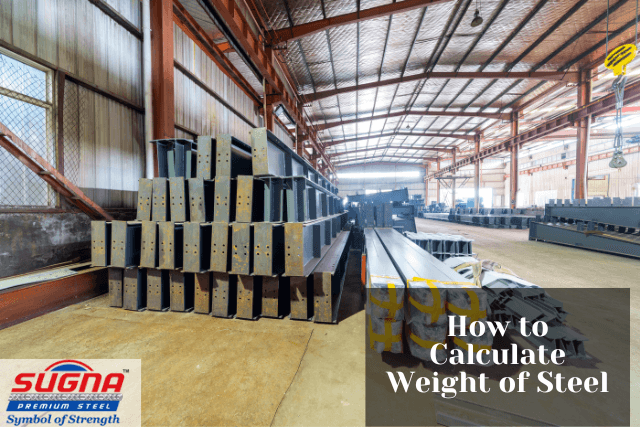Stronger Than The Strongest TMT

1800 1036 176
040- 2766 3527

##### WhatsApp

+91 86390 37981# How to Calculate the Weight of Steel?If you are buying steel or any kind of steel products, either for building construction or otherwise, there are certain things that you must always be aware of. One such most important thing that you should know while buying steel is how to calculate weight of steel

While using steel and construction materials made of steel, you’ll find yourself in many situations where you would want to know the weight of the steel you are using. It can be for the sake of billing, procurement, or determining utilization. In fact, weight also plays an important role in determining the quality of steel.

Further, most of the engineering and construction products made of steel are sold by the measure of weight only. This includes products such as steel pipes, TMT bars, plates, steel wires, etc. Therefore, it becomes even more important for both the buyers and the sellers to know how to calculate the weight of steel accurately and reliably.

However, worry not. In this article, we are going to give away to you all the details on how to calculate the weight of steel – accurately, and reliably. We also share with you a mathematical equation or a formula that will help you calculate the weight of steel, almost instantaneously. This calculation uses the steel weight calculation formula. Now, let’s go step by step, so read on!

## Parameters Affecting Steel Plate Weight

One does not have to be a genius to know that not all types of steel would weigh the same. The weight of a steel bar or product depends upon multiple factors. Therefore, to calculate the weight of steel, we would need to have this information about the steel we are looking at.

Like any calculation, this equation also has multiple variables. It has two variables, or factors, to be precise.

These parameters are:

1. Material Dimension
2. Material Density

Before proceeding further to the actual calculation, let us understand these parameters first.

• ### Material Dimension (Length, breadth, thickness)

The dimensions of the steel bar, rod, or pipe that you want to measure the weight of are the most important piece of primary information that you would require to understand how to calculate weight of steel bar. The primarily includes the length, breadth, and thickness of the steel.

Therefore, whenever you want to measure the weight of the steel, be sure to measure and note down its dimensions first. Once you have this information handy, it becomes easier to plug these figures into the formula while doing the calculations.

Note: It is important to make sure that all three dimensions (length, breadth, thickness) are expressed in the same units of measurement (inch, cm, mm, etc) and not different.

• ### Material Density

The second most important parameter that you need to determine before you can figure out how to calculate weight of steel sheet is its density. Different types of steel can have different densities and hence the weight also varies.

As a rule of thumb, the weight of the steel product is directly proportional to the density of the steel used to make it. In other words, a steel product with higher density will weight more than a similar product with similar dimensions, but made with low-density steel. This explains why certain steel rods which have the same dimensions, look the same but have different weights.

Generally, if we look at carbon steel, its density lies somewhere around 7850 Kg/m3. If you are measuring stainless steel, it will have a density of around 8000 Kg/m3. However, please note that these densities can vary greatly depending on the make, quality, grade, etc of the steel that you are using.

## How to determine the density of your steel?

The density of the specific grade of steel is standardized according to the ASTM design standards, which all good manufacturers of TMT steel such as SUGNA TMT follow. Moreover, you can also refer to the specific design codes to determine the density of the steel, or reach out to the manufacturer directly to enquire about the steel density.

Sugna TMT, which is one of the top manufacturers of TMT steel bars in the region, always provides complete information to its clients about the quality of steel, including steel density.

As weight is proportional to density, once you get the density and values of 3 dimensions (Length, Breath, and Thickness), you can use the formula for calculating the weight of steel. So, let’s move toward the formula.

## The formula for Calculating the Weight of SteelNow, that you know the parameters required for measuring the weight of steel, and how and where to determine them, let us now tell you about the primary question at hand – that is, the steel weight calculation formula

So, here it is:

Formula to calculate steel weight (W) with Length (L); Breadth (B), Thickness (T), and Density (D)

Weight of Steel plate =Density of the plate * Volume of the plate

W = D * ( L * B * T )

Where,

W = Weight of the steel plate

L = Length of the steel plate

B = Breadth of the steel plate

T = Thickness of steel plate

D = Density of the steel plate

The metric units (units of measurement) that should ideally be used here are:

• Kg (Kilograms) for weight,
• Kg/m3 (Kilograms per cubic meter) for density, and
• L, B, and T in m (meters).

The above units will help you determine how to calculate weight of steel bar in kg.

## Disclaimer:

At the time of purchase, the actual weight of the metal may show a significant variation from the weight that you would calculate using this steel weight calculation formula. This is completely okay and can be attributed to the variations of tolerance and composition during manufacturing.

## Conclusion

The answers to the questions such as How to calculate weight of steel bar, How to calculate weight of steel rod, and How to calculate weight of steel sheet are fairly simple and easy process using the steel weight calculation formula

Good TMT manufacturers such as Sugna TMT will help you determine the weight of your product, with complete transparency. So, do not forget to use this knowledge, and be assured of the weight of the steel while purchasing it from a top manufacturer such as Sugna TMT.